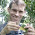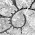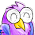## Thursday, November 8, 2012

### Mapping the reconstructed value of a quantitative character on the tree

Following my new function, densityMap, for mapping the posterior density for a discrete character from stochastic mapping on three tree (1, 2), it seemed natural to do a quantitative trait version. In this case, we just compute the ML ancestral character states at the nodes under some model (say, BM); fraction the ends into many small pieces; interpolate the states along all the edges; and then map the ancestral states to a color scheme. Note that I am interpolating using equation (3) from Felsenstein (1985), which I believe will give me the correct set of internal states. I have programmed this in a new function, contMap (continuous trait mapping), which I will soon post to the phytools page.

Here's a quick demo:
> library(phytools)
> source("contMap.R")
> tree<-pbtree(n=30)
> x<-fastBM(tree)
> contMap(tree,x,lwd=6,res=200,legend=1.5)
Pretty cool.

We can also try a "real" example. Here is body size (log SVL) in Greater Antillean anoles:
> contMap(anoletree,svl,fsize=c(0.7,1))

1.This probably should have been my 300th post. Oh well.

2.Liam, this is really nice! Summarizing ancestral state reconstructions of a continuous trait in a non-abrupt way has always been challenging, but this solves that problem.

1.Thanks Graham.

3.Hmm. Is there anyway to map uncertainty in a similar way; maybe by smoothing out the range of per-node confidence intervals across the branches?

1.Hi David. Not sure, but I have been thinking about similar things. Liam

4.Hi Liam.
I'm wondering whether is it possible to invert colours used in this function, e.g. the largest values red and the lowest values blue? It seems to me as a more logical way. I was trying to do it myself, as it seemed easy, just invert the scale of "cols", though I got stuck on "object 'getState' not found". Would you mind give me a clue what to do?
Ivana

5.Dear Liam, I hace a problem when I tried to map continuous character on my tree this is the ouput with the error message

b

Phylogenetic tree with 10 tips and 9 internal nodes.

Tip labels:
CDM, a49, a40, a41, a42, a46, ...

Rooted; includes branch lengths.

> x<-fastBM(b)
> contMap(b,x,lwd=6, plot=T, type="phylogram", legend=T)
Error en while (x > trans[i]) { :
valor ausente donde TRUE/FALSE es necesario

What I'm doing wrong..?..thanks!

1.Does your branch have any branches of zero length or very short branches. This is known to cause a problem for the function. You can do:

b<-di2multi(b)
contMap(b,x)

Let us know if this solves the problem. If it doesn't it may be that you have very short internal edges or terminal edges (tip branches) of zero length. Very short internal edges you can set to zero and then use multi2di; short terminal edges you can lengthen to give slightly non-zero length, e.g.:

tree\$edge.length[which(tree\$edge.length==0)]<-1e-6

for example.

- Liam

2.This is true, my tree have some branches of 0 length. I modify It but I still but unsuccessfully

b\$edge.length[which(b\$edge.length==0)]<-1e-6
> x<-fastBM(b)
> contMap(b,x)

Error en tree\$maps[[i]] <- XX[, 2] - XX[, 1] :
se dan más elementos que los que pueden ser sustituídos

3.Hi Marcelo.

Not sure. Why don't you send me your tree file and I will troubleshoot?

All the best, Liam

4.6.Hi Liam and Marcelo,

I am dealing with the same error.

Error in tree\$maps[[i]] <- XX[, 2] - XX[, 1] :
more elements supplied than there are to replace

What I am doing here is first transform the branch lengths of a phylogeny using the alpha parameter I obtained from a OU fit. Then, I want to perform an ancestral state reconstruction. It is true that, the transformation with the OU alpha makes some internal nodes close to zero, but even using "di2multi" I keep obtaining the same error. Please can you post how you solve this problem?

Many thanks,

Regards,

Oscar

phy.OU<-transform(phylo, model="OU", 0.127)

phy.OU<-di2multi(phy.OU)

trait_ancestral<-contMap(phy.OU, trait.vec, res=100, lwd=1, outline=T, fsize= 0.3)

Error in tree\$maps[[i]] <- XX[, 2] - XX[, 1] :
more elements supplied than there are to replace

1.Hi Oscar. Try increasing tol in multi2di. tol is the shortest branch length that is considered to be "zero length".

Eventually, I will fix this bug. Sorry for the inconvenience. Note if you plot the OU tree the branch lengths will be stretched according to your OU model, which you may not want.

All the best, Liam

7.Thanks Liam for your prompt response

Adding this line did the job

phy.OU<-di2multi(phy.OU, tol=1e-01)

Best,

Oscar

8.Hi Liam,
I seem to be running into the same issue with contMap, I keep getting the 'Error in tree\$maps[[i]] <- XX[, 2] - XX[, 1] :
more elements supplied than there are to replace' message. Increasing tol in multi2di doesn't seem to do the trick. Any other suggestions for how to solve this problem? Any insights appreciated.
cheers

1.Actually Liam, solved it by increasing the res value. Please ignore the previous query.
cheers

9.I may be missing something obvious here, but is there a way to use the very nice plotting function utilized in contMap to plot data for a tree where the states at nodes have already been estimated? Thanks!

1.Not presently, Ben. Great idea.

One of the problems is that contMap estimates the states along the edges of the tree (as well as at nodes). (It does this using an equation from Felsenstein to interpolate - but this is equivalent to what we would get at any intermediate point along the edge if we had a node there.) If you have ancestral state estimates from an alternative model of evolution, then interpolating in this way becomes invalid.

One alternative is to use plotBranchByTrait & then just color each edge by the state at the parent or daughter node (or their arithmetic mean). This is not too difficult to do & you can probably find demos on the blog; however you lose the continuous color gradient of contMap.

- Liam

2.Thanks Liam,

Will check try plotBranchbyTrait. An alt might be to associate ancestral state with node labels then plot with FigTree- but will be a bit awkward to do I think...Hope to see something along these lines implemented in phytools at somepoint in the future!

Thanks, Ben

3.Ok, sorry for the multiple questions: now relating to plotBranchbyTrait- how do the nodes need to be labelled in the vector of states:

is it using makeNodeLabel from ape (start labeling nodes from 1 at root)

or as in phytools when you
> plot(tree)
>nodelabels()
(start labeling at root from 1+no of tips).

Have tried both of these but am getting an error message:
from:

>plotBranchbyTrait(tree, test, mode="nodes")

Error in while (p >= breaks[i] && p > breaks[i + 1]) i <- i + 1 :
missing value where TRUE/FALSE needed

Can get plotBranchbyTrait to work fine for tips...Thanks in advance,

Ben

Ben

4.Think I have figured this out:

traits need to be a vector of tip values AND node values, with node labeling following phytools, rather than ape naming.

5.Hi Ben.

Yes - I'd forgotten this myself, but it looks like if mode="nodes" then the vector x should be in order of the node numbers in tree\$edge - that means 1:N (for N tips) should be the tip values in order tree\$tip.label, and (N+1):(N+m), for m internal nodes, should be the nodes in the order of tree\$edge. Let me know if this makes sense!

- Liam

6.Hi Liam,

Yes thats what I did. In the end, I found the simplest way to make a pretty gradient shaded tree was to use the writeAncestors() function, then pop the tree into FigTree. Not very elegant but it works!

Cheers,

Ben

10.Hi Liam

I am running into the same problem as a few people commenting here, namely Error in tree\$maps[[i]] <- XX[, 2] - XX[, 1] :

I have tried all the solutions presented here, and none of them have worked. In addition, I have 3 trees and trait sets, all of which are sub-trees of one larger tree. One of these works fine with contMap, but the other two return the error I mentioned above. Also, the one that works has a polytomy in it and works without first using multi2di, the two that fail also have polytomies, yet fail.

Any help would be appreciated!

Henry

11.I had the same error as some of the others here:

Error in while (x > trans[i]) { : missing value where TRUE/FALSE needed

My phylogeny was fine; it worked when I used simulated data (fastBM). The problem was that contMap needed a named vector (which you, in your defense, state explicitly in the documentation).

So I did as follows:

named.vector<- sorteddata\$CVbm
names(named.vector) <- ultrametric.tree\$tip.label
contMap(ultrametric.tree,named.vector,lwd=2,res=200,legend=.5, fsize=.6)

...which worked. Thought I'd share in case someone else has the same problem.

Btw Liam, thanks for getting the last round in Seville. It was fun; hope to meet you at another conference, and I'll get you back.

Jostein

1.Well, not the same error as the others, but AN error...

12.I Am Usually To Blogging And I Actually Appreciate Your Content. The Article Has Actually Peaks My Interest. I Am Going To Bookmark Your Web Site And Maintain Checking For New Information. judi online

13.Dear Liam. How can I visualize a tree with 182 tips? I mean, how can I make "zoom" in the plot generated? Thanks, Alicia

Note: due to the very large amount of spam, all comments are now automatically submitted for moderation.